# Multiengine Aircraft Weight and Balance Computations

Weight and balance computations for small multiengine airplanes are similar to those discussed for single-engine airplanes. See Figure 1 for an example of weight and balance data for a typical light twin-engine airplane.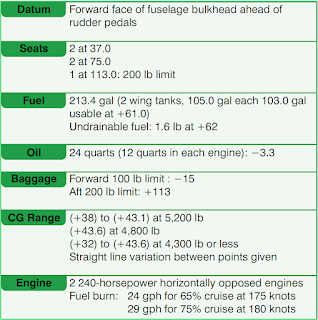Figure 1. Typical weight and balance data for a light twin-engine airplane

The airplane in this example was weighed to determine its basic empty weight (BEW) and empty weight center of gravity (EWCG). The weighing conditions and results are:

Weight with fuel drained and oil full:
Right wheel scales: 1,084 lb, tare 8 lb
Left wheel scales: 1,148 lb, tare 8 lb
Nose wheel scales: 1,202 lb, tare 14 lb

First, add the weights indicated by the individual scales and then subtract the tare weights to determine the BEW. Next, using the BEW and EWCG, the loaded weight and CG of the aircraft can be determined with data from Figure 2, using a chart such as the one in Figure 3.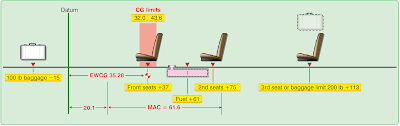Figure 2. Twin-engine airplane weight and balance diagram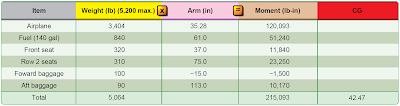Figure 3. Determining the loaded CG of the sample airplane in Figure 2

The aircraft is loaded as shown:
Fuel (140 gal):  840 lb
Front seats: 320 lb
Row 2 seats: 310 lb
Forward baggage: 100 lb
Aft baggage: 90 lb

### Chart Method Using Weight, Arm, and Moments

Make a chart showing the weight, arm, and moments of the airplane and its load.

The loaded weight for this flight is 5,064 pounds, and the CG is located at 42.47 inches aft of the datum.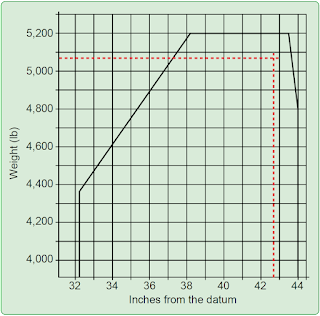Figure 4. Sample CG range chart

To determine that the weight and CG are within the allowable range, refer to the CG range chart in Figure 4. Draw a line vertically upward from 42.47 inches from the datum and one horizontally from 5,064 pounds. These lines cross inside the envelope, showing that the airplane is properly loaded.

### Determining the CG in Percentage of Mean Aerodynamic Chord (MAC)

Refer again to Figures 2 and 3.
The loaded CG is 42.47 inches aft of the datum.
The MAC is 61.6 inches long.
The LEMAC is located at station 20.1.
The CG is 42.47 – 20.1 = 22.37 inches aft of LEMAC.

Use the formula in Figure 5 to find the CG in percent MAC.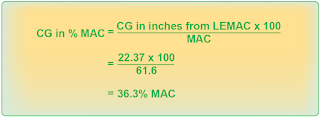Figure 5. Finding CG in percent MAC

The loaded CG is located at 36.3 percent MAC.

### The Chart Method Using Weight and Moment Indexes

As mentioned in the Single-Engine Aircraft Weight and Balance Computations section, anything that can be done to make careful preflight planning easier makes flying safer. Many manufacturers furnish charts in the Pilot’s Operating Handbook/Aircraft Flight Manual (POH/AFM) that use weight and moment indexes rather than weight, arm, and moments. The charts also help reduce errors by including tables of moment indexes for the various weights.

Cruise fuel flow = 16 gallons per hour
Estimated time en route = 2 hours, 10 minutes
Reserve fuel = 45 minutes = 12 gallons
Total required fuel = 47 gallons

The pilot completes a chart like the one in Figure 6 using moment indexes from tables in Figures 7 and 8.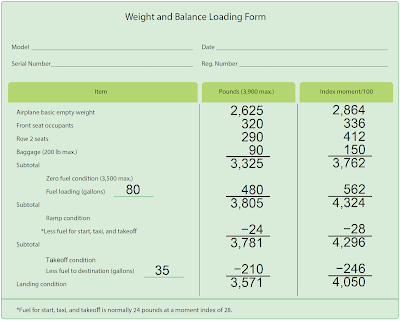Figure 6. Typical weight and balance loading form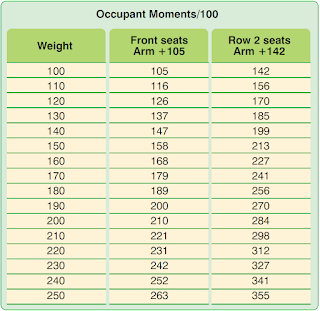Figure 7. Sample weight and moment index for occupants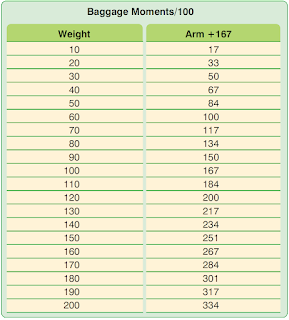Figure 8. Sample weight and moment index for baggage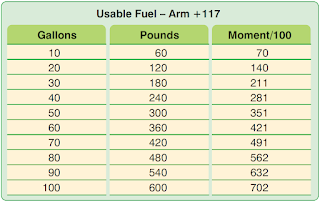Figure 9. Sample weight and moment index for fuel

The moments divided by 100 in the index column are found in the charts in Figures 7 through 9. If the exact weight is not in the chart, interpolate between the weights that are included. When a weight is greater than any of those shown in the charts, add the moment indexes for a combination of weights to get that which is desired. For example, to get the moments divided by 100 for the 320 pounds in the front seats, add the moment index for 100 pounds (105) to that for 220 pounds (231). This gives the moment index of 336 for 320 pounds in the front seats.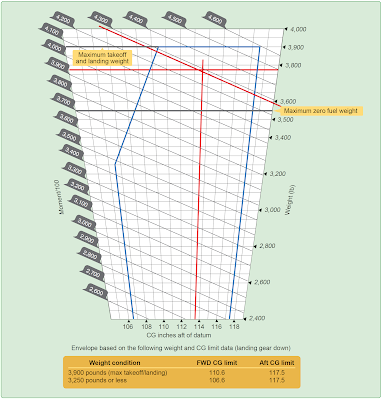Figure 10. Sample moment limits versus weight envelope

Use the moment limits versus weight envelope in Figure 10 to determine if the weight and balance conditions are within allowable limits for both takeoff and landing at the destination. The moment limits versus weight envelope is an enclosed area on a graph of three parameters. The diagonal line representing the moment divided by 100 crosses the horizontal line representing the weight at the vertical line representing the CG location in inches aft of the datum. When the lines cross inside the envelope, the aircraft is loaded within its weight and CG limits.

Takeoff: – 3,781 lb and 4,296 moment divided by 100
Landing: – 3,571 lb and 4,050 moment divided by 100

Locate the moment divided by 100 diagonal line for 4,296 and follow it down until it crosses the horizontal line for 3,781 pounds. These lines cross inside the envelope at the vertical line for a CG location of 114 (113.6) inches aft of the datum.

The maximum allowable takeoff weight is 3,900 pounds, and this airplane weighs 3,781 pounds. The CG limits for 3,781 pounds are 109.8 to 117.5. The CG of 114 (113.6) inches falls within these allowable limits.

RELATED POSTS